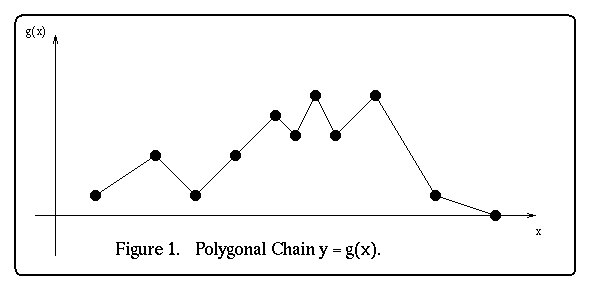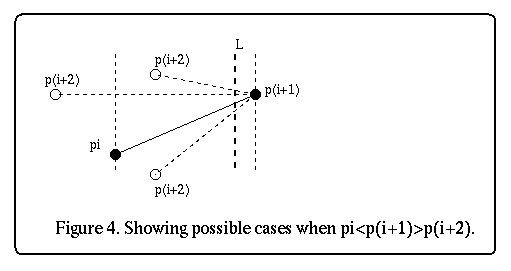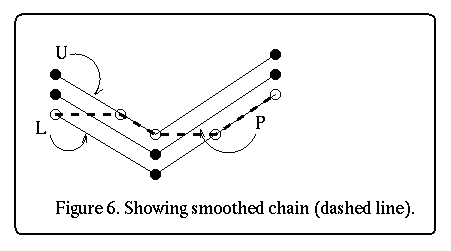# Hysteresis Smoothing for X-Monotonic Polygonal Chains# 1. Introduction

Usually in some applications, the system is driven by some external input which could be an output of another system/process. In most cases this output contains noise, and before it is applied, smoothing is performed on it to remove most of the noise. The input considered in this report will be thought of as a single- valued one dimensional function, g(x). The function g(x) can be thought of as a polygonal chain in the x - y=g(x) plane (see figure 1).In this report we will look at one such method of smoothing called hysteresis smoothing. Hysteresis smoothing is a method of smoothing polygonal chains such that local minima/maxima within a certain threshold value t is smoothed out (removed).
This is how it is done :
Let P = be a polygonal chain monotonic in the x direction. Let t be the threshold value.
Form a chain U = <u1,...,un>, which is an exact copy of P but translated by t/2 in the positive y direction. Also let L = <l1,...,ln> be an exact copy of P but translated by t/2 in the negative y direction. See figure 2 below.Lastly, connect u1 and l1 to p1, un and ln to pn, to form a monotonic polygon in
the x direction, B = <l1, p1, u1,...,un,pn,ln,...,l1>. The smoothed chain, S, (see figure 2) is produced as follows: First we rotate the polygon B by 90 degrees so that it becomes monotonic in the y direction. Now, imagine that l1 provides a continuous supply of water and trace the path of the resulting waterfall inside the polygon B (u1 could have done as well). The path of the waterfall is the hysteresis-smoothed chain.

## 2. Hysteresis Smoothing Algorithm

We will discuss only the algorithm for polygonal chains monotonic in the x direction. A polygonal chain is monotonic in the x direction if every vertical line intersecting it does so in at most one point or one line segment (see figure 3(a) and 3(b)). This implementation is for a polygon B monotonic in the x direction (it was not rotated by 90 degrees!).Notation
P = denotes the input polygonal chain with its vertices labeled       from left to right.
p(i).x or pi.x --- is the x coordinate of pi, and
p(i).y or pi.y --- is the y coordinate of pi.
t = threshold.
SEG(pi, pj) = Line segment between points pi and pj.

Algorithm
1. If p1.x <= p2.x <= ... <= pn.x is NOT satisfied the reject and stop.
P is not monotonic in the x direction.

2. Form the upper chain U = <(p1.x,p1.y + t/2),...,(pn.x,pn.y + t/2)> and
the lower chain L = <(p1.x,p1.y + t/2),...,(pn.x,pn.y + t/2)>.

3. STACK Q <-- empty;
POINT T, V, Last;

T <-- l1;
PUSH(Q,l1);
WhichChain <-- L;
Last.x <-- ln.x;
Last.y <-- l1.y;
T_changes <-- FALSE;
For> i := 2 to n-1 Do
BEGIN (* for *)

3.1         If li.y = T.y
{
PUSH(Q,li);
WhichChain <-- L;
T_changes <-- TRUE;
}
3.2         If ui.y = T.y
{
PUSH(Q,ui);
WhichChain <-- U;
T_changes <-- TRUE;
}
3.3         If li.y > T.y
If l(i-1).y < T.y (* there is proper intersection *)
{
V <-- Intersection point of SEG(l(i-1), li) and y=T.y;
PUSH(Q,V);
PUSH(Q,li);
WhichChain <-- L;
T_changes <-- TRUE;
}
Else
{
PUSH(Q,li);
WhichChain <-- L;
T_changes <-- TRUE;
}
3.4         If ui.y < T.y
If u(i-1).y > T.y (* there is proper intersection *)
{
V <-- Intersection point of SEG(u(i-1), ui) and y=T.y;
PUSH(Q,V);
PUSH(Q,ui);
WhichChain <-- U;
T_changes <-- TRUE;
}
Else
{
PUSH(Q,ui);
WhichChain <-- U;
T_changes <-- TRUE;
}
3.5         If (WhichChain = L) AND T_changes
{
T <-- li;
Last.y <-- li.y;
}
Else If T_changes
{
T <-- ui;
Last.y <-- ui.y;
}
T_changes <-- FALSE;
END (* for *)
PUSH(Q,Last);
S = Q;
End (* algorithm *)

# 3. Complexity and Correctness

Complexity

The test for monotonicity (step 1) can easily be done in O(n) time by a while loop.
Step 3 is a simple for loop, and all the statements in the body, including computing the intersection point can be done in constant time. Note also that only the push operation is performed on the stack. Thus the total time complexity of the algorithm is O(n).

Correctness
In step 1, if pi.xp(i+2) then P is not monotonic in the x direction. Note that the vertices of P are labeled from left to right. Figure 4 shows what happens.In step 3, the algorithm checks all of the following cases (figure 5):A moment's thought reveals that this are the only possible cases worth checking. Thus, when the algorithm terminates, the stack Q contains all the points of the smoothed chain, S, with the first point pushed being s1 and the last pushed being sk (2 <= k <= 2n -1). The last two points may be identical, but this does not pose any problems. Note that S may contain more points than the original chain P, see figure 6. Clearly, this algorithm does not perform data reduction as its primary goal and may not be useful in applications which data reduction is critical. Also, extending this algorithm to non-monotonic polygonal chains is not trivial.# 4. Using the Applet

Inputting Polygonal Chains and The Threshold
Points are input by clicking the left mouse button in the applet's area. They are joined automatically by line segments in the order they were input. Clicking the middle or right mouse button signals the end of input. The threshold value t is entered by using the scroll bar at the top of the applet's area. The default value for t is 25 units.

Running The Algorithm
Press the Compute button to run the algorithm on the input chain. The RED chain is the resulting hysteresis-smoothed chain.

To test different values of the threshold t on the same polygonal chain, press the Clear New Chains button to delete all the new chains displayed except the input chain. Then change the threshold by using the scroll bar and running the algorithm again.Home Page